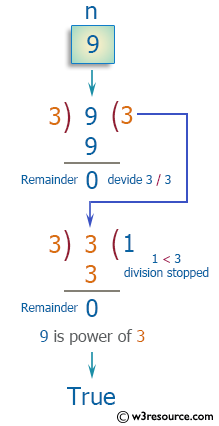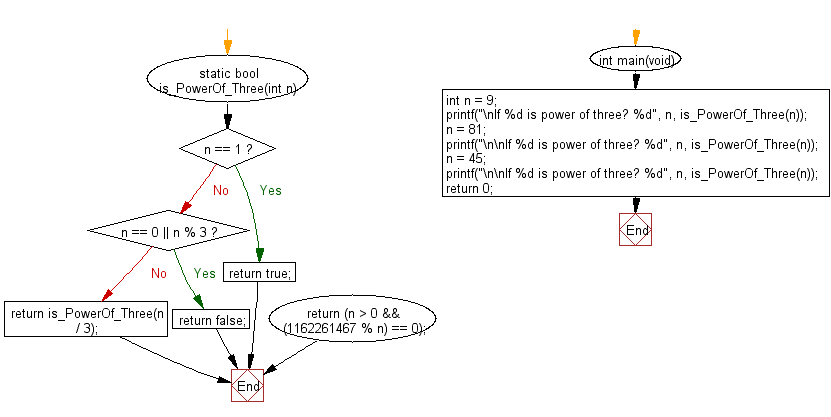﻿ C Program: Check if a given integer is a power of three - w3resource

# C Exercises: Check if a given integer is a power of three

## C Programming Mathematics: Exercise-13 with Solution

Write a C program to check if a given integer is a power of three.

Example:
Input: 9
Output: true
Input: 81
Output: true
Input: 45
Output: false

Pictorial Presentation:Sample Solution:

C Code:

``````#include <stdio.h>
#include <stdbool.h>

static bool is_PowerOf_Three(int n) {
#if 0
if (n == 1) return true;
if (n == 0 || n % 3) return false;
return is_PowerOf_Three(n / 3);
#else
return (n > 0 && (1162261467 % n) == 0);
#endif
}
int main(void)
{
int n = 9;
printf("\nIf %d is power of three? %d", n, is_PowerOf_Three(n));
n = 81;
printf("\n\nIf %d is power of three? %d", n, is_PowerOf_Three(n));
n = 45;
printf("\n\nIf %d is power of three? %d", n, is_PowerOf_Three(n));
return 0;
}
```
```

Sample Output:

```If 9 is power of three? 1

If 81 is power of three? 1

If 45 is power of three? 0
```

Flowchart:C Programming Code Editor:

Improve this sample solution and post your code through Disqus.

What is the difficulty level of this exercise?

Test your Programming skills with w3resource's quiz.

﻿